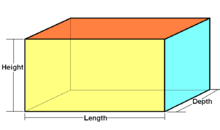# The Packaging Boxfaiyaz26Intra NSU Programming Con...
Limits 1s, 512 MB

VHL is an international mail service company. Customers can send various packages to different places of the world via them.

VHL is planning to make a standard package box to contain the client's documents and stuff.

And they want to use different paper in different side of the box. And each paper costs different or maybe same.

The paper costs $P$, $Q$ and $R$ per square meter for the bottom, sides and the top respectively.

The box have to have a total volume of $V m^3$. Check the figure below:The company wants to have the dimensions in a way such that the total cost of construction is as low as possible.

Now you need to write a program that will take $P$, $Q$, $R$ and $V$ as input and output the minimum cost of building the box.

## Input

Input starts with a positive integer $T$ ($≤ 500$), denoting the number of test cases.

Each case starts with a line containing four real numbers denoting $P$, $Q$, $R$, $V$.

Each value is at least 1.0 and at most $10^4$ and contains at most five places after the decimal point.

And $P$, $Q$, $R$ will be distinct.

## Output

For each line of input produce a line of output. Each line will contain one real number denoting the minimum cost to build the box. Errors less than $10^{-6}$ will be ignored.

## Sample

InputOutput
1
1 2 5 96

288.00000000


For the $\text{height}=6$, $\text{depth} = 4$, $\text{length = 4}$, we get the minimum cost of 288.TernarySearchuDebug

### Submit